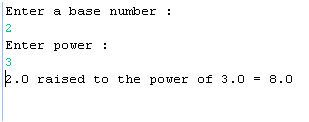# Java Math.pow() Example

This example explains you about the pow() method in Java. This tutorial explains you about the Math.pow() method using a simple example. In this example you can see that how can we find the power of a number in Java. Power means the exponent of a number i.e. n^p (n raised to the power of p) for example if the expression has written like 2^3 then it should be calculated as 2*2*2.## Java Math.pow() Example

In this section we will discuss about how to get the power of a number in Java.

This example explains you about the pow() method in Java. This tutorial explains you about the Math.pow() method using a simple example. In this example you can see that how can we find the power of a number in Java. Power means the exponent of a number i.e. n^p (n raised to the power of p) for example if the expression has written like 2^3 then it should be calculated as 2*2*2.

Example

Here an example is being given which will demonstrate you about finding out the power of a number. In this example we will use the pow() method of Math class. To accomplish this task we will create a Java class where we will write the code for calculating the power of a number. In this example we will write the code for taking the number from command line.

Source Code

GetPowerExample.java

```package desktop;

import java.io.*;
public class GetPowerExample {

public static void main(String args[])
{
try
{
System.out.println("Enter a base number : ");

System.out.println("Enter power : ");

double result = Math.pow(n, p);
System.out.println(n+" raised to the power of "+p+" = "+result);
}
catch(Exception e)
{
System.out.println(e);
}
}
}
```

Output

After executing above example successfully a message will be prompt on console for providing the base number, when you will input the number and press enter then another message will be prompt for input the power when you will press the enter result will be displayed as follows :